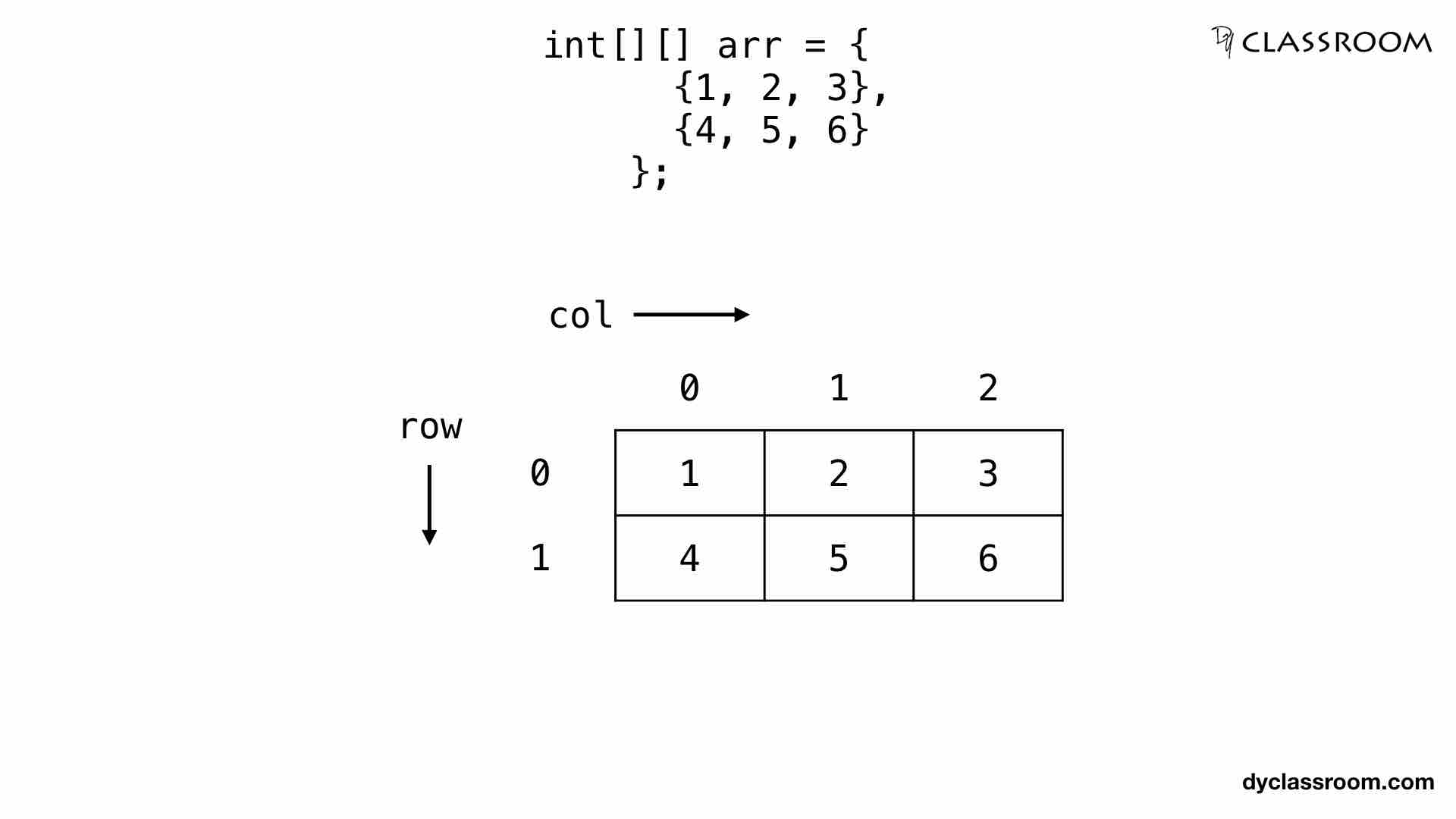# Java - Two Dimensional Arrays

JavaIn this tutorial we will learn about Two Dimensional Arrays in Java programming language.

We use two dimensional, 2D array to store data in rows and columns format.

## Declaring a 2D array

Following is the syntax to declare a 2D array.

``````dataType[][] arrayName;
``````

Where, name of the array variable is `arrayName` and its data type is `dataType`.

We can also declare 2D array in the following manner.

``````dataType arrayName[][];
``````

This is similar to C programming language.

In the following example we are declaring a 2D array `arr` of data type `int` to store integer values.

``````int[][] arr;
``````

Now, lets go ahead and create a 2D array.

## Creating a 2D array

To create a 2D array we use the `new` keyword as shown below.

``````arrayName = new dataType[row_size][col_size];
``````

Where, `arrayName` is the name of the array variable of data type `dataType` and it has `row_size` number of rows and `col_size` number of columns.

In the following example we are creating an array `arr` having 2 rows and 3 columns and of data type `int`.

``````arr = new int;
``````

## Declaration and Creation of 2D array

In the following example we are declaring and creating a 2D array `arr` of having 2 rows and 3 columns and of data type `int`.

``````int[][] arr = new int;
``````

## Assigning and Accessing value of a 2D array

In the following example we are creating a 2D array `arr` having 2 rows and 3 columns and of data type `int`.

To access the element of the array we are using the row-index and column-index like `arr[r][c]` to access element at rth row and cth column.

``````class Array2DExample {
public static void main(String args[]) {
// create an array
int[][] arr = new int;

// int variable
int count = 1;

// use for loop to assign value to an array
for (int r = 0; r < 2; r++) {
for (int c = 0; c < 3; c++) {
arr[r][c] = count;
count++;
}
}

// print the value of the array
for (int r = 0; r < 2; r++) {
for (int c = 0; c < 3; c++) {
System.out.print("arr[" + r + "][" + c + "] = " + arr[r][c] + " ");
}
System.out.println();
}
}
}
``````

Output:

``````arr = 1 arr = 2 arr = 3
arr = 4 arr = 5 arr = 6
``````

Another way of assigning the values is by using `{}` curly brackets and using comman separated values.

``````class Array2DExample {
public static void main(String args[]) {
// create an array
int[][] arr = {
{1, 2, 3},
{4, 5, 6}
};

// print the value of the array
for (int r = 0; r < 2; r++) {
for (int c = 0; c < 3; c++) {
System.out.print("arr[" + r + "][" + c + "] = " + arr[r][c] + " ");
}
System.out.println();
}
}
}
``````

We can even assign value index-wise like the following.

``````class Array2DExample {
public static void main(String args[]) {
// create an array
int[][] arr = new int;

// assign value to 1st row having r-index 0
arr = 1;
arr = 2;
arr = 3;

// assign value to 2nd row having r-index 1
arr = 4;
arr = 5;
arr = 6;

// print the value of the array
for (int r = 0; r < 2; r++) {
for (int c = 0; c < 3; c++) {
System.out.print("arr[" + r + "][" + c + "] = " + arr[r][c] + " ");
}
System.out.println();
}
}
}
``````

## Representation of 2D array

The following image depicts a 2D array `arr`.

``````// array having 2 rows and 3 columns
int[][] arr = {
{1, 2, 3},
{4, 5, 6}
};
``````## Example #1: Write a Java program to find the average score of two students in three papers

Given, score of first student is 60, 55 and 70 while score of the second student is 80, 60 and 41.

We can store the score of the two students in a 2D array having 2 rows and 3 columns. The rows will represent the student and the columns will hold the score of the students.

``````class Array2DExample {
public static void main(String args[]) {
// create an array
int[][] score = {
{60, 55, 70},
{80, 60, 41}
};

// sum array
int[] sum = new int;

// sum of 1st student
sum = 0;

// sum of 2nd student
sum = 0;

// average array
float[] avg = new float;

// compute sum
for (int r = 0; r < 2; r++) {
for (int c = 0; c < 3; c++) {
sum[r] += score[r][c];
}
}

// compute average of 1st student
avg = (float)sum / 3;

// compute average of 2nd student
avg = (float)sum / 3;

// print result
System.out.println("Average score of 1st student = " + avg);
System.out.println("Average score of 2nd student = " + avg);
}
}
``````

Output:

``````Average score of 1st student = 61.666668
Average score of 2nd student = 60.333332
``````